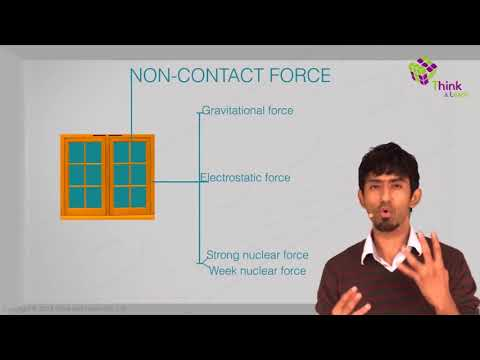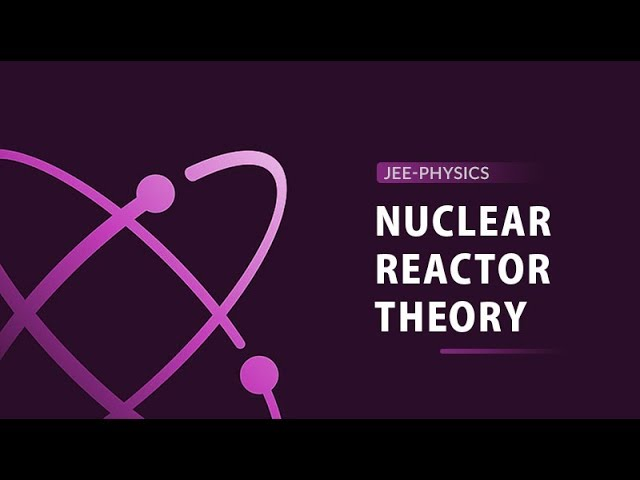# Nuclear Physics

Nuclear physics is the branch of physics that studies atomic nuclei and their constituents and interactions. Examples of nuclear interactions or nuclear reactions include radioactive decay, nuclear fusion and fission. In this article, let us study nuclear physics, nuclear physics theory, nuclear force, and radioactivity in detail.

## What is Nuclear Physics?

Nuclear physics is a scientific discipline that studies the structure of nuclei, their formation and stability. It mainly focuses on understanding the fundamental nuclear forces in nature and the complex interactions between neutrons and protons.

Nuclear Physics is defined as the branch of physics deals with the structure of the atomic nucleus and its interactions.

Experimental nuclear physics drives innovation in scientific instrumentation. Today’s research in nuclear physics is enabling a range of new technologies in materials science chemistry, medicine, and biology. The application of nuclear physics lies largely in the field of power generation using nuclear energy. Once the force holding the nucleus was understood, we started splitting and fusing neutrons. The process of splitting the nucleus to generate energy is known as Nuclear Fission and the process of fusing two neutrons to generate energy is known as Nuclear Fusion.

## Top 15 Most Important and Expected Questions on Nuclei in Hindi.## Nuclear Physics Theory

In this section, we have discussed the fundamentals of atomic nuclei:

‘R’ represents the radius of nucleus.

 $$\begin{array}{l}\large R = R_{o}\;A^{\frac{1}{3}}\end{array}$$

Where,

• Ro is the proportionality constant
• A is the mass number of the element

### Total Number of Protons and Neutrons in a Nucleus

The mass number (A), also known as the nucleon number, is the total number of neutrons and protons in a nucleus.

 A = Z + N

Where,

• N is the neutron number
• A is the mass number
• Z is the proton number

### Mass Defect

Mass defect takes place when some mass is lost during the nuclei generation.

 $$\begin{array}{l}\large \Delta m = Z\,m_{p}+(A-Z)m_{n}-M\end{array}$$

Where,

• M is the mass of the nucleus
• $$\begin{array}{l}\Delta m\end{array}$$
is the difference between the mass of the nucleons and mass of the nucleus
• $$\begin{array}{l}m_{p}\end{array}$$
is the Mass of the Proton
• $$\begin{array}{l}m_{n}\end{array}$$
is the mass of the Neutron

### Packing Fraction

Packing fraction is defined as Mass defect per nucleon.

Packing fraction (f) = Mass defect per nucleons

$$\begin{array}{l}\large Packing\;fraction (f) = \frac{\left [ Zm_{p}+(A-Z)m_{n}-A \right ]}{A}\end{array}$$

### Nature of Nuclear Force

The nuclear force acts between the charges and functions as the gravitational force between masses. Nuclear force acts between the protons and neutrons of atoms. Nuclear force is much stronger than the Coulomb force. The nuclear physics is based on the forces known as nuclear force. The nature of nuclear force is given as:

• Nuclear forces are attractive in nature.
• These forces are independent of charges.
• The range of nuclear forces is short.
• As the distance between two nucleons reduces, the nuclear force becomes weak between them.
• The nuclear force is dependent on the spin.

Here is a video for you! The video describes the nuclear force with examples explaining its properties.## Difference Between Nuclear Physics and Atomic Physics

The main difference between nuclear physics and atomic physics is that nuclear physics deals with the nucleus while atomic physics deals with an entire atom. More specifically, atomic physics deals with the atom as a system consisting of a nucleus and electrons. Nuclear physics deals with the nucleus as a system consisting of nucleons (protons and neutrons).

Atomic physics concerns itself with the entire atom and how the electronic configuration of electrons can change. When an atom loses an electron, it becomes positively charged (cations) and when it gains an electron it becomes negatively charged (anions).

Radioactivity is a nuclear process that occurs due to the decay of the nucleus. Radioactivity is based on the law of conservation of charge. External parameters such as temperature and pressure do not affect the rate of decay.

The daughter nuclei will have unique physical and chemical properties (that is different from parent nuclei). The decay rate of any radioactive material is directly proportional to the number of atoms present at that instant. α, β, and γ rays are followed during the radioactivity.

There are two units of radioactivity and they are:

• Curie (Ci): If the radioactive substance decay at the rate of 3.7×1010 decays per second, then the unit used is Curie.
• Rutherford (rd): If the radioactive substance decay at the rate of 106 decays per second, then the unit is Rutherford.

## Applications of Nuclear Physics

Some important applications of nuclear physics are listed below:

• Nuclear energy is an essential part of society’s energy production. Nuclear energy is produced using a nuclear reactor.
• Nuclear physics principles have led to great medical diagnostics and therapy methods. One such example is radiation therapy. In radiation therapy, ionizing radiation is used to treat conditions such as thyroid cancer.
• In archaeology, nuclear solutions are used to identify various stable and radioactive isotopes in archaeological relics. This allows us to uncover the backgrounds of these artefacts.
• Radioactive elements are used in smoke detectors. Most standard domestic smoke alarms use a radioactive isotope of the element americium to recognize smoke.

## Solved Example on Nuclear Physics

Example 1:

Consider a radioactive material which is undergoing fusion and resulting in a mass defect of 2 kg. Calculate the amount of energy released from the material.

Solution:

Mass defect, m = 2 kg

Velocity of light, c = 3 х 108 m/s

Using Einstein’s equation,

E = mc2

E = 2 х (3 х 108)2

E = 1.8 х 1017 J

 Related Articles:

See the video below and learn about the nuclear reactor.## Frequently Asked Questions – FAQs

Q1

### What is nuclear physics?

Nuclear Physics is defined as the branch of physics deals with the structure of the atomic nucleus and their interactions.

Q2

### Who is the father of nuclear physics?

Ernest Rutherford is the father of nuclear physics.

Q3

### What is the use of nuclear physics?

Nuclear energy is an essential part of society’s energy production. Nuclear energy is produced using a nuclear reactor. In archaeology, nuclear solutions are used to identify various stable and radioactive isotopes in artefacts.
Q4

Radioactivity is a nuclear process that occurs due to the decay of the nucleus. Radioactivity is based on the law of conservation of charge.

Q5

### What are the units of radioactivity?

The following are the units of radioactivity:

Curie, Becquerel and Roentgen are the units of radioactivity.

## Watch the video and study Modern Physics JEE PY QuestionsTest your Knowledge on Nuclear Physics